# Python Strings

Strings are contiguous set of characters in between quotation marks. You are free to use either pairs of single or double quotes to use the strings in your python program.

## Python String Example

Here is an example of creating string in python:

```str1 = 'Hello Python'
str2 = "This is Python Strings Tutorial"```

To print the above created string as output. Then here is the code:

```# Python Strings - Example Program

str1 = 'Hello Python'
str2 = "This is Python Strings Tutorial"

print(str1, "\n", str2);```

Here is the output produced by the above python program:Here is another example, demonstrates the concept and use of string in python:

```# Python String - Example Program

str = 'Hello Python'

print (str)             # this will print the complete string
print (str)          # this will print the first character of the string
print (str[2:8])        # this will print the characters starting from 3rd to 8th
print (str[3:])         # this will print the string starting from the 4th character
print (str * 3)         # this will print the string three times
print (str + "String")  # this will print the concatenated string```

Here is the sample output of the above python program:Here is another example of string, this is loopy string program.

This example shows how to ask from the user to enter his/her name or any other word and print each letter of the word one by one.

```# Python String Example
string_var = input("Enter your name:");
print("Following are the letters one by one, used to form",string_var);
for all_letter in string_var:
print(all_letter);```

Here is the sample output of loopy string example code given above in Python.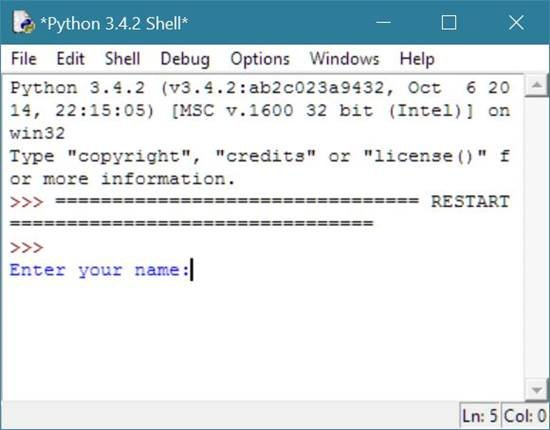Now enter your name, for example, codescracker here, and press ENTER button to see the output like in the screenshot given below.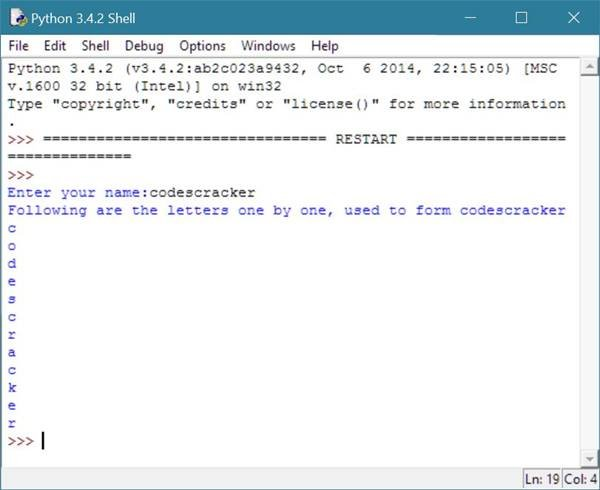Let's take another example shows how to find length of the string given by or entered by the user using the len() function.

```# Python String Example
string_msg = input("Enter a string:");
print("\nLength of the entered string is",len(string_msg));```

Here is the sample output of the above string example in python.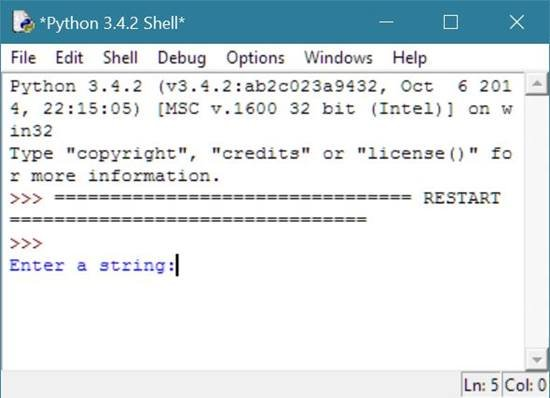Now enter any string, here i am going to enter python string at codescracker and press the ENTER button to see the following output.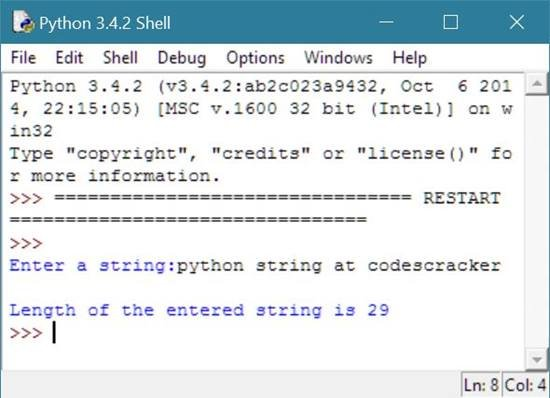Let's take an example shows how to ask from the user to enter any string and remove all vowels from the string and then print the same string without any vowels in python.

```# String example in python
# removing vowels from string
string_with_vowels = input("Enter a string containing vowels:");
string_without_vowels = "";
VOWELS = "aeiou";
print("\nRemoving all the vowels from the given string..");
for each_letter in string_with_vowels:
if each_letter.lower() not in VOWELS:
string_without_vowels += each_letter;
print("All vowels are successfully removed from the string.");
print("\nNow the string is:\n",string_without_vowels);```

Below is the sample output after running the above string example code in python.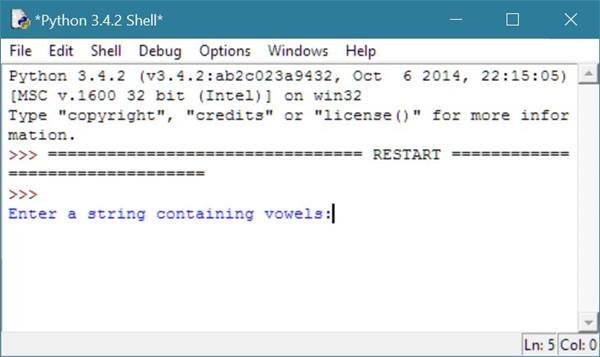Now enter the string say, This is python at codescracker and press enter to see the following output.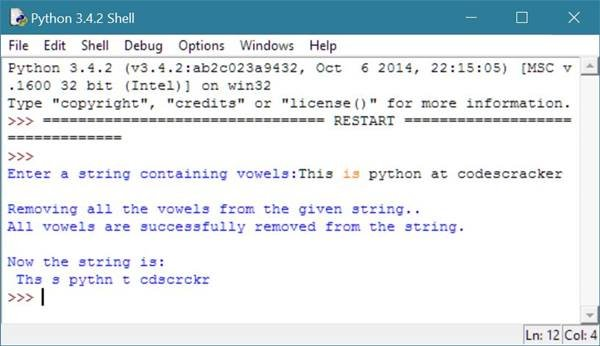Now let's modify the above example with following code to print the string without vowel along with removed vowels from the same string.

```# String example in python
string_with_vowels = input("Enter a string containing vowels:");
string_without_vowels = "";
VOWELS = "aeiou";
vowels_in_string = "";
print("\nRemoving all the vowels from the given string..");
for each_letter in string_with_vowels:
if each_letter.lower() not in VOWELS:
string_without_vowels += each_letter;
else:
vowels_in_string += each_letter;
print("All vowels are successfully removed from the string.");
print("\nNow the string after removing all vowels is:\n",string_without_vowels);
print("\nAnd vowels removed from string is:\n",vowels_in_string);```

Here is the output you will see, after doing or typing the same string and pressing the enter button.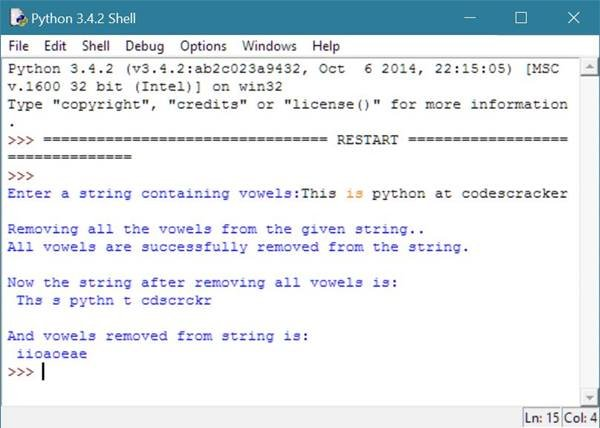## Python Escape Sequence Characters

Here the following table lists the escape sequence character code and their name available in python:

Escape Sequence Character Code Output
\\ Print one backslash
\' Prints a single quote
\" Prints a double quote
\b Moves cursor back one space
\a Alert Bell
\cx Control-x
\b Backspace
\C-x Control-x
\f Formfeed
\e Escape
\M-\C-x Meta-Control-x
\r Carriage return
\n Newline
\nnn Octal notation
\s Space
\x x
\t Tab
\v Vertical tab
\xnn Hexadecimal notation

## Python Escape Sequences Example

Here is an example of escape sequence with string in Python.

```# This is Python Escape Sequence with String Example
print("\t\t\tPython String Tutorial");
print("\t\t\t \\ \\ \\ \\ \\ \\ \\");
print("\t\t\t\tby");
print("\t\t\tcodescracker.com");
print("\t\t\t \\ \\ \\ \\ \\ \\ \\");
print("\nThanks to all Python programmer,");
print("who are visiting codescracker.com to improve their skills.");
```

Here is the sample run of the above python string example code of escape sequence:## String Concatenation in Python

String concatenation means the addition of two string. You can concatenate two string using the + operator in Python.

Let's take an example demonstrates string concatenation in Python.

```print("Let me clear about " + "the string concatenation in Python");
print("String concatenation in Pyt" + "hon can easily be done");
print("just by using the plus or '+' operator\n");
print("You can also concatenate \
string spread in multiple" + " \
line into one single \
"+ "\
string as output \
just by using the \
backslash or '\' operator \
in Python");
```

When you run the above example code in Python of string concatenation using the F5 function key, you will see the following output.## Repeating String in Python

You can easily repeat string in Python using * operator as shown in the example given below.

```# String Repeating Example in Python
print("This is String repeat in Python.");
print("Below code will repeat the string stringRepeat 10 times\n");

print("stringRepeat" * 10);

print("\nBelow code will repeat the string stringRepeat 10 times with space\n");

print("stringRepeat " * 10);```

Here is the sample run of the above string repeating example code in Python.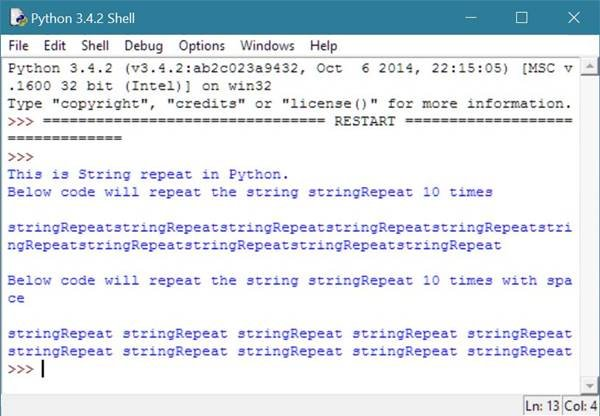## Python Indexing String

Indexing of string can easily be done in Python language.

Here is an example shows how to index a string in python.

```# Python String Example
# This example shows how to index string in python
import random;
string = input("Enter any string:");
print("The entered string is",string);
for k in range(len(string)):
pos = k;
print("String[",pos,"]\t",string[pos]);```

Here is the sample output after running the above string example code of indexing string in python.Now enter any string say codescracker and press enter to see the output given below: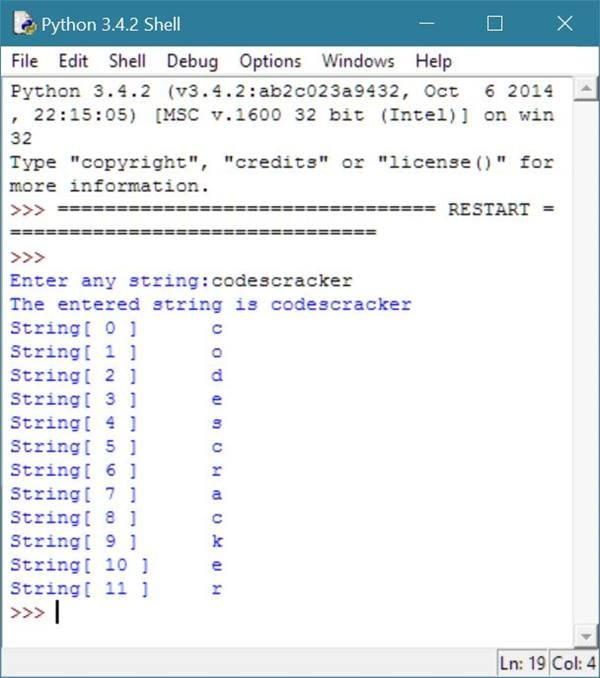Now let's modify the above string indexing example with random access of string indexing program in python.

```# Python String Example
# Indexing string in python example
# Python random access example program
import random;
string = input("Enter any string:");
print("The entered string is",string);
highest_len_value = len(string);
lowest_len_value = -len(string);
for k in range(10):
pos = random.randrange(lowest_len_value, highest_len_value);
print("String[",pos,"]\t",string[pos]);```

Let's see the output after entering the same string, that is, codescracker in this case.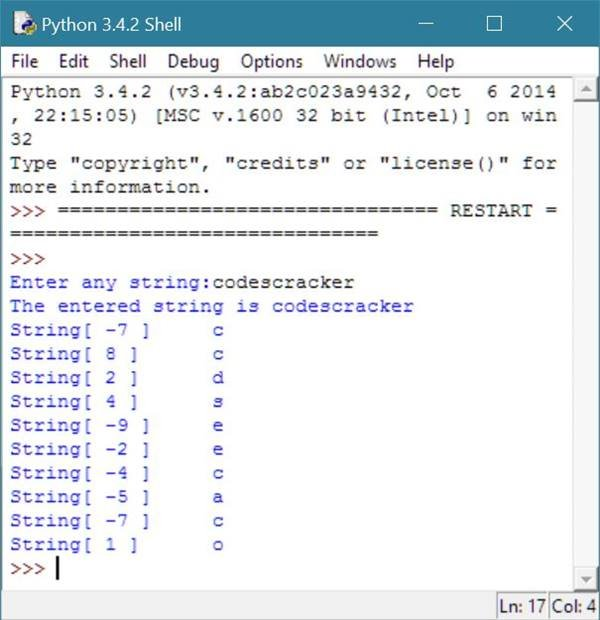You can also index and print the string's character using the below example.

First initialize the string say codescracker to any variable say string like

`string = "codescracker";`

as shown in the screenshot given below: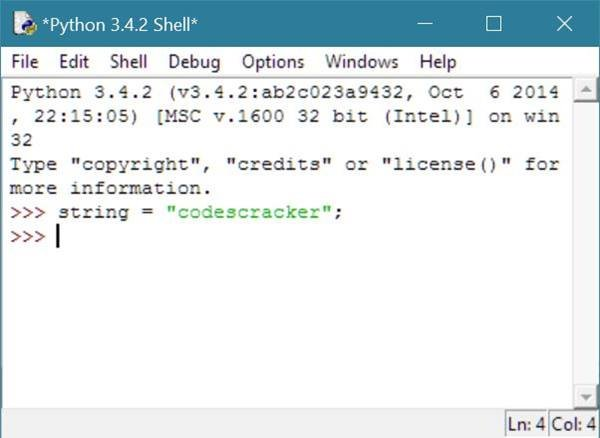Now, write the code like

`print(string);`

will give c as output. Or

`print(string);`

will give o as output. Or

`print(string);`

will give d as output and so on, as shown in the screenshot given below.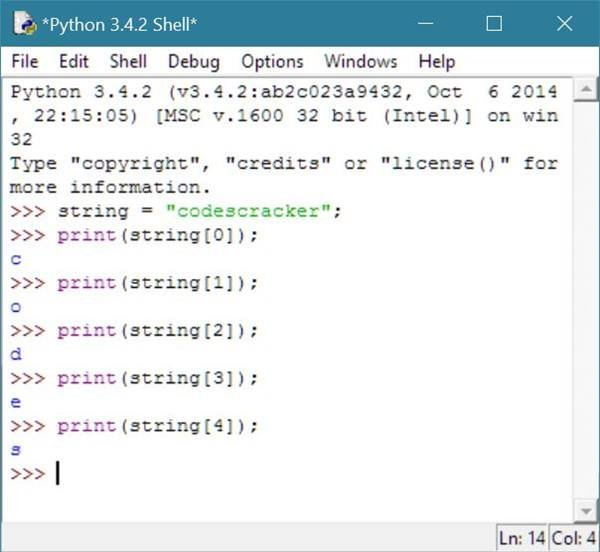Or, write the code like

`print(string[-1]);`

will give r as output. Or

`print(string[-2]);`

will give e as output. Or

`print(string[-3]);`

will give k as output. Or

`print(string[-10]);`

will give d as output. Or

`print(string[-12]);`

will give c as output and so on, as given in the following screenshot.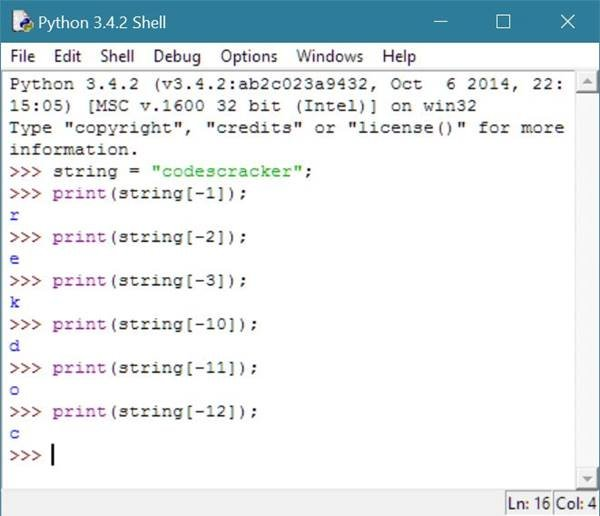## Python Slicing Strings

Let's take an example to understand how string slicing works in python.

Here in this example, program will ask from the user to enter any string say codescracker. Then ask to enter the starting and ending index no. of the string entered by user, here codescracker. And when user press ENTER button, then the character/string is sliced out from the string according to provided beginning and ending index no. of the string.

For example, the table given below lists the character/string according to its starting and ending index number in python. Here in this case, and variable is string and the string is codescracker is assigned to the variable.

Starting Index No. Ending Index No. Python Code to Slice String Output
0 12 string[ 0 : 12 ] codescracker
1 11 string[ 1 : 11 ] odescracke
2 10 string[ 2 : 10 ] descrack
4 8 string[ 4 : 8 ] scra
-12 12 string[ -12 : 12 ] codescracker
-12 -1 string[ -12 : -1 ] codescracke
-12 2 string[ -12 : 2 ] co

and so on.

Now let's take the example of string slicing in python.

```# String example in python
# Slicing string example in python
# String slicer program in python
string = input("Enter any string:");
print("To slice the string, enter beginning and ending index no. of the string.");
print("To exit, press ENTER without providing the index no.");
begin_index = None;
while begin_index != "":
begin_index = input("\nEnter Begin Index No.:");
if begin_index:
begin_index = int(begin_index);
end_index = int(input("Enter End Index No.:"));
print("string[",begin_index,":",end_index,"] = ",string[begin_index:end_index]);```

Here is the sample output of above string slicing program in python.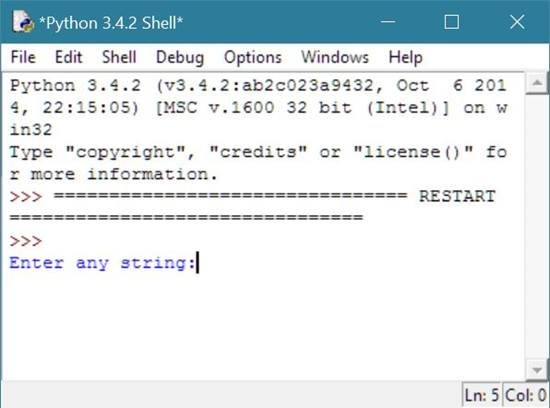Now enter the string, codescracker here.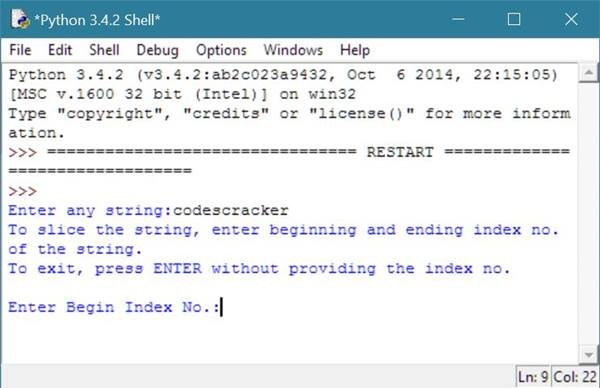Now start entering the beginning and ending index number to find out the sliced string as shown in the output given below.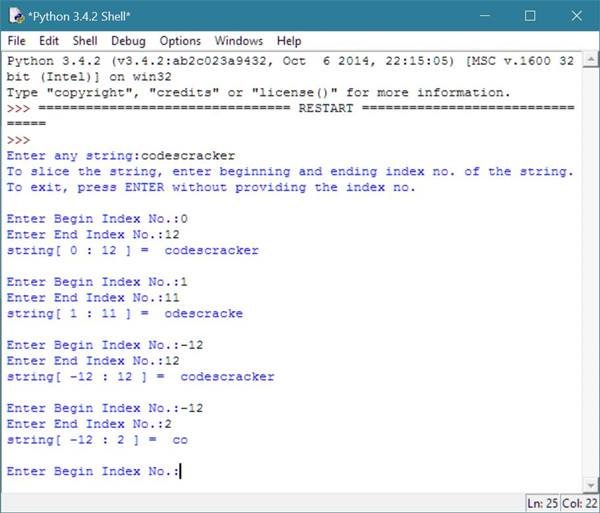## Python Popular String Methods

The table given below list down some popular and useful methods related to Python string with its short description.

String method Description
upper() returns string into uppercase form
lower() returns string into lowercase form
title() returns first letter of the string in uppercase and rest of the string into lowercase form
replace(old string, new string) returns same string just after replacing old string with new string value

Let's take an example demonstrating all the string methods in Python as given in the above table.

```# Python String Methods Example
original_string = "You are learning String in Python at codescracker";
print("Original String:", original_string);
print("String in uppercase:", original_string.upper());
print("String in lowercase:", original_string.lower());
print("String as title:", original_string.title());
print("String with replacement:", original_string.replace("in Python", "Methods in Python"));
print("String with replacement:", original_string.replace("in Python", "Methods"));
print("Original string is still:", original_string);```

Here is the sample output of the above string methods example code in Python.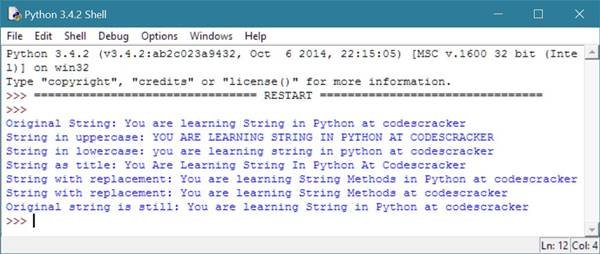### More Examples

Here are the list of some more examples on strings in python:

Tools
Calculator

Quick Links
Signup - Login - Give Online Test